# LOG#088. Group theory(VIII).Schur’s lemmas are some elementary but very useful results in group theory/representation theory. They can be also used in the theory of Lie algebras so we are going to review these results in this post (for completion).

FIRST SCHUR’S LEMMA. If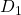andare 2 finite-dimensional irreducible representations of G, and if A is certain linear map (generally a matrix) fromtosuch as it commutes with the action of the group (for any element), i.e.,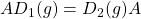then at least one of the following consequences holds:

1) A is invertible, and then the representation are necessarily equivalent.

2) A=0.

SECOND SCHUR’S LEMMA. If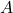is a complex matrix of orderthat commutes with every matrix from an irreducible representationThen, A must be a scalar matrix multiple of the identity matrix, i.e.,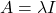.

Schur’s lemmas and their corollaries are used to prove the so-called (Schur’s) orthogonality relations and to develop the basics of the representation theory of finite groups. Schur’s lemmas admits some generalizations to Lie groups and some other kind of structures, such as Lie algebras and other sets of operators or matrices. Therefore, it is important to understand Schur’s lemmas!

Consequences of these lemmas:

1) Irreducible representations of an abelian finite group are one dimensional.

2) Orthonormality of group representations. Letbe a finite group, and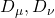two irreducible representations of G. Then,3) Completeness of irreducible representations. Letbe a finite group and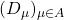the set of every irreducible representation of G. Then,4) The number of irreducible representations of a finite group is finite and they can be computed with the aid of the following formula:We can give some elementary examples of irreducible representations of abelian (finite) groups.

1) For, thenand so there are two and only two irreducible representations, both one dimensional: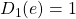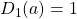2) For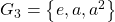, then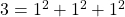and there are 3 irreducible representations:

1st. The simplest representation, given by2nd. The next possible representation is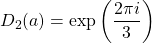3rd. The last representation is provided by: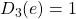3) For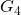we have two cases:and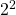. And then we have:

3.1) The case of the cyclic group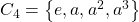with 4 unidimensional representations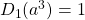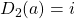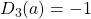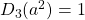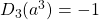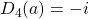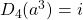3.2) The case of the Klein group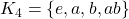where,andwith. The representations of this group, in the unidimensional case, are given by: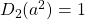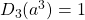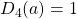Of course, you can build the matrix representation of the above group representations as a nice homework. :).

This site uses Akismet to reduce spam. Learn how your comment data is processed.